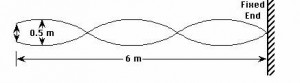Courses

# Test: Standing Waves

## 5 Questions MCQ Test Physics Class 11 | Test: Standing Waves

Description
This mock test of Test: Standing Waves for JEE helps you for every JEE entrance exam. This contains 5 Multiple Choice Questions for JEE Test: Standing Waves (mcq) to study with solutions a complete question bank. The solved questions answers in this Test: Standing Waves quiz give you a good mix of easy questions and tough questions. JEE students definitely take this Test: Standing Waves exercise for a better result in the exam. You can find other Test: Standing Waves extra questions, long questions & short questions for JEE on EduRev as well by searching above.
QUESTION: 1

### A node is a point where there is always

Solution:

A node is a point along the medium of no displacement. The point is not displaced because destructive interference occurs at this point.

QUESTION: 2

### A cylindrical tube, open at both ends, has a fundamental frequency f in air. The tube is dipped vertically in water so that half of it is in water. The fundamental frequency of the air-column is now:

Solution:

As we know, f=v/2l
Now, it will act as one end and one end closed.
So, f0=v/2l’=v/4½=v/2l=f

QUESTION: 3

### Fundamental note in open pipe (v1 = ν/2L) has _________ the frequency of the fundamental note in closed organ pipe (v2 = ν/4L).

Solution:

Let L be a length of the pipe,
The fundamental frequency of closed pipe is
v2​=ν​/4L                                         .....(i)
where ν is the speed of sound in air.
Fundamental frequency of open pipe of same length is
v​1=ν/2L​                                            .....(ii)
After dividing v1 with v2,
v1/v2= ν/2L/ ν​/4L
v1=2v2

QUESTION: 4

Equation of longitudinal stationary waves in the pipe is

Solution:
QUESTION: 5

In an experiment to find the speed of waves in a rope, a standing wave pattern is established as shown in diagram below. The vibrating end of rope makes 90 vibrations per minute. The speed of the waves isSolution:

If there are exactly 90 vibrations in 60.0 seconds, then there is a frequency of 1.5 Hz. The diagram shows 1.5 waves in 6.0-meters of rope; thus, the wavelength is 4.0 meters. Now use the equation v=f*w to calculate the speed of the wave. Proper substitution yields 6.0 m/s.